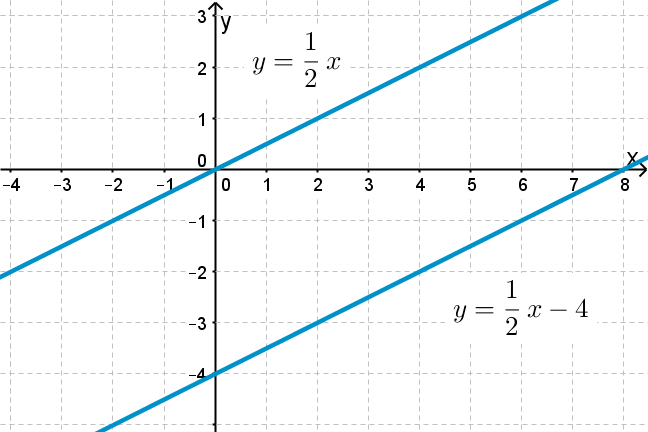## Want to keep learning?

This content is taken from the UNSW Sydney's online course, Maths for Humans: Linear and Quadratic Relations. Join the course to learn more.
1.13

## UNSW Sydney# Transformations of lines

We now apply translations and dilations to lines, or more precisely to the equations of those lines. What happens to the equation of a line if we translate it by $$\normalsize{2}$$ in the $$\normalsize{x}$$ direction, or by $$\normalsize{-3}$$ in the $$\normalsize{y}$$ direction? What happens if we apply a dilation $$\normalsize{[x,y]}$$ goes to $$\normalsize{[rx,ry]}$$?

In this step you will learn

• how a translation in either the $$\normalsize{x}$$ or $$\normalsize{y}$$ direction affects the equation of a line

• how a dilation affects the equation of a line.

## Translating a line

If we take the line $$\normalsize{y=3x-1}$$ and translate it up by $$\normalsize{2}$$ in the $$\normalsize{y}$$-direction, we get $$\normalsize{y=3x-1+2}$$ or just

$\Large{y=3x+1.}$

If we take the same line $$\normalsize{y=3x-1}$$ and translate it by $$\normalsize{2}$$ in the $$\normalsize{x}$$ direction, then the equation changes more subtly to $$\normalsize{y=3(x-2)-1}$$. Can you see why? Suppose the point $$\normalsize{[r,s]}$$ lies on the original line. This means that

${\Large s = 3r - 1 = 3( (r+2)-2) - 1}.$

But then $$\normalsize{[r+2,s]}$$ will lie on the translated line $${\normalsize y = 3(x-2)-1 = 3x-7}$$.

Q1 (E): Which of the following equations represents a translation of $$\normalsize{y=3x-1}$$ by $$\normalsize{3}$$ in the negative $$\normalsize{x}$$ direction?

a) $$\normalsize{y=3(x-3)-1}$$
b) $$\normalsize{y=3(x+3)-1}$$

Q2 (M): Find the equation of the red line, and hence find the equation of its translate the blue line.Summarizing, we have the following:

Replacing $$\normalsize{y}$$ by $$\normalsize{y-k}$$ in an equation represents a translation by $$\normalsize{k}$$ in the $$\normalsize{y}$$ direction.

Replacing $$\normalsize{x}$$ by $$\normalsize{x-h}$$ in an equation represents a translation by $$\normalsize{h}$$ in the $$\normalsize{x}$$ direction.

## Scaling a line

If we multiply the $$\normalsize{y}$$ coordinate of a point $$\normalsize{[x,y]}$$ which lies on the line $$\normalsize{y=3x-1}$$ (in red) by $$\normalsize{\frac{3}{2}}$$, the effect is a dilation in the $$\normalsize{y}$$ direction by just this factor. This takes the line $$\normalsize{y=3x-1}$$ to $$\normalsize{y=\frac{9}{2}x-\frac{3}{2}}$$, which is the blue line below.If we multiply the $$\normalsize{x}$$ coordinate of a point $$\normalsize{[x,y]}$$ on the line $$\normalsize{y=3x-1}$$ by $$\normalsize{\frac{1}{2}}$$, the effect is a dilation in the $$\normalsize{x}$$ direction by just this factor.Summarizing, we have the following:

Replacing $$\normalsize{y}$$ by $$\normalsize{ry}$$ in an equation represents a dilation by $$\normalsize{\frac{1}{r}}$$ in the $$\normalsize{y}$$ direction.

Replacing $$\normalsize{x}$$ by $$\normalsize{sx}$$ in an equation represents a dilation by $$\normalsize{\frac{1}{s}}$$ in the $$\normalsize{x}$$ direction.

## Combining translation and dilation

So what happens if we combine a translation by $$\normalsize{3}$$ in the $$\normalsize{y}$$ direction with a dilation by $$\normalsize{4}$$ in the $$\normalsize{x}$$ direction? The line $$\normalsize{y=3x-1}$$ first goes to $$\normalsize{(y-3)=3x-1}$$ and then to $$\normalsize{(y-3)=3(x/4)-1}$$. This works out to be $$\normalsize{y=(3/4)x+2}$$.A1. The translate by $$\normalsize{-3}$$ in the $$\normalsize{x}$$ direction is b) $$\normalsize{y=3(x+3)-1}$$. This simplifies to the equation $$\normalsize{y=3x+8}$$.
A2. The point $$[0,5]$$ lies on the red line, and so its equation is of the form $$y=kx+5$$ for some number $$k$$. Since the point $$[-3,-4]$$ also lies on this line, this becomes $$y=3x+5$$. The blue line is the translate in the $$x$$ direction of the red line by $$\normalsize{4}$$, and therefore has equation $$y=3(x-4)+5$$ or $$y=3x-7$$.